This video was brought to you by GeneralPAC.com, making power systems Intuitive, Open and Free for Everyone, Everywhere. Consider subscribing and supporting through patreon.com/GeneralPAC. This is a mechanism for you to support us financially so we can continue making high quality power system video tutorials. Our corporate sponsor for this topic is AllumiaX.com from Seattle, Washington. Contact them for industrial and commercial power system studies.

Introduction to the Delta Wye transformer connection Part 5c.

In this part, we'll continue to do a phasor analysis and look at what the relationship is between line current and phase current. In the last part, we looked at the phasor analysis between line current A and phase current A and phase current C – and saw that the line current A, compared to the phase current A, there's a magnitude difference of the square root of 3, and there's a phase difference of 30 degrees between these two currents.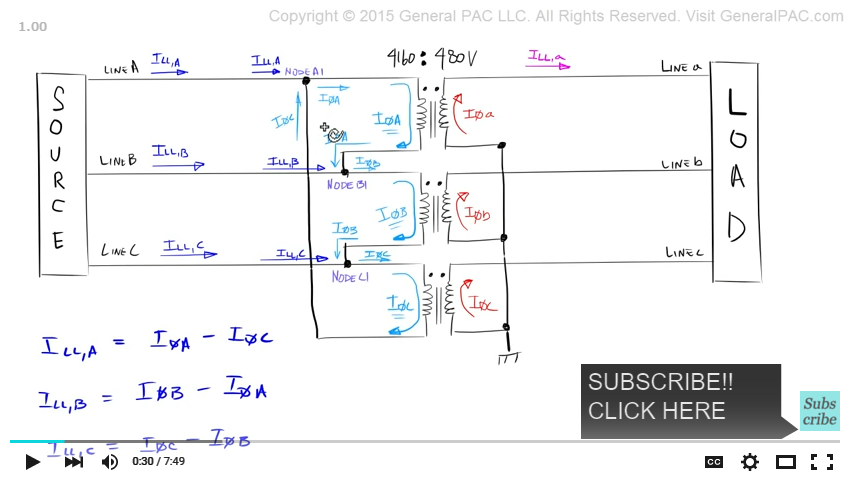So in this video, let's look at the relationship between line current B and phase current B – and see if we get the same relationship. Okay So – what we're going to analyze – is this particular equation. So we know that line current B which is going into the winding B. Phase current B minus phase current A – which is coming from winding A. Now the reason why the equation is setup like this – is because our delta connection is setup in this particular fashion. Where the polarity side of winding B is connected to node B1 and the non-polarity side of winding A is also connected to node B1.

Let's evaluate this equation. Alright – so we have a balanced system like we did like in our previous example. This is what we did in the previous example. I'm going to put phase C back to where it was. So here is phase A – it's at the 0 degrees mark and it's defined by this particular equation. Here is phase B it's at the 240 degree mark and it's defined by this particular equation.  And here is phase C – which is at the 120 degree mark and it's defined by this equation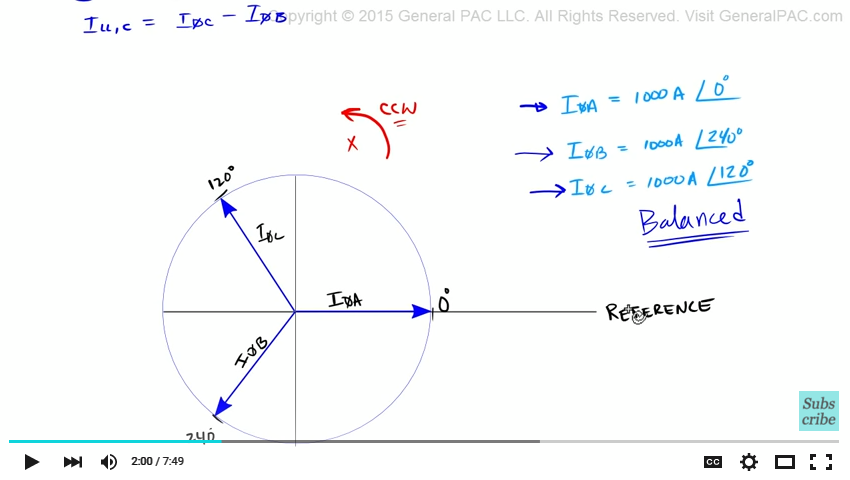Now this is what we call a balanced system – and there's dedicated video that talks about this setup. Okay – so we're going to evaluate this equation here. Line current B is equal to phase current B minus Phase current A. So what we're going to do is – we're going to pick up phase current A and add it to the head of phase current B. Now the resultant vector would equal phase current B plus phase current A.

But that's not what we want – we want phase current B minus phase current A. So we would have to take phase current A – pick that up and rotate it 180 degrees- and that would equal negative phase current A. So our resultants vector would start at the origin – and end at the tip of negative phase A current. And that resultant vector equals phase current B minus phase current A – and that essentially equals line current B.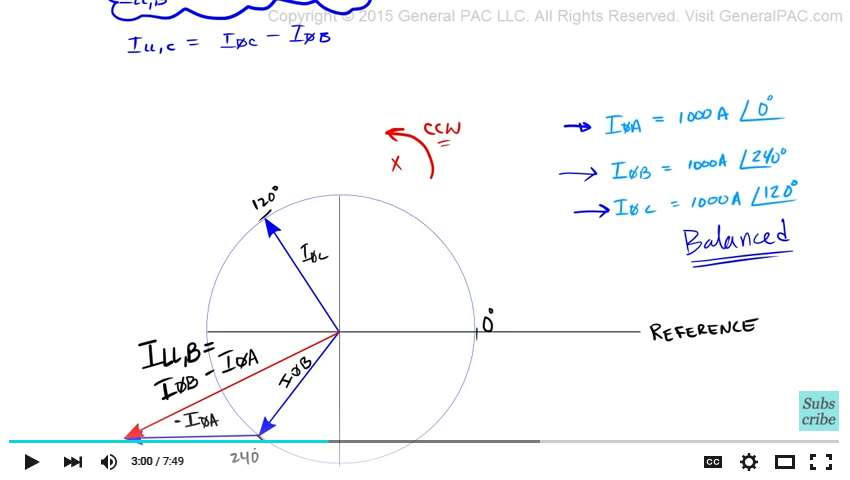Right? So our line current B – the magnitude of line current B is the square root of 3 times phase current B – very similar to our last example in part 5b right? And our line current B is also 30 degrees lagging compared to phase current B.  Now it's lagging because all of the phasors - we assumed that they are all rotating in the counter clockwise direction. Phase current B is ahead of line current B right? As they rotate in the counter clockwise direction. So line current B lags phase current B by 30 degrees. So 30 degrees is the phase difference between those two phasors right? So very similar to part 5b.

Let's evaluate this equation very quickly. Our line current C which is this current here – is equal to phase current C which is the current that's going to going to phase C winding. Minus phase current B which is the current that's coming winding B and into node c1.. you see that?

Alright so we're going to evaluate this particular equation now. So what we'll do is –we'll leave this line current B as it is but we'll make a copy of phase B and we'll put here at the head of phase current C but we'll have to rotate it 180 degrees because it's negative phase current B. So this right here – we're going to label this negative phase current B.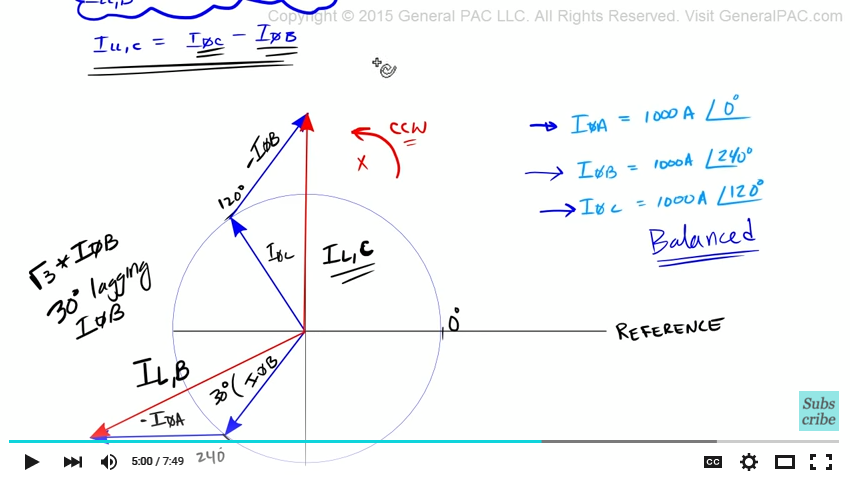The resultant vector is going to start at the origin and end at this tip here. Now our resultant vector equal phase current C minus phase current B. Now comparing it to phase current C – our resultant vector which is our line current – line current C. Our resultant vector is the square root of 3 larger than phase current C and it also has a 30 degrees phase shift compared to phase current C.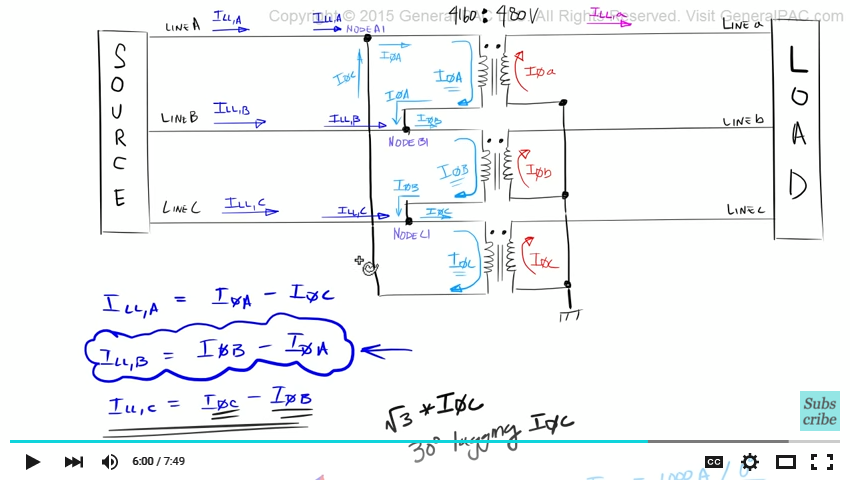Alright you see that? As they all rotate in the counter clock direction, there's a 30 degrees phase shift between phase C current and line current C. Okay so what we're saying essentially is that line current C which is going into node C1. If we compare line current C with phase current C, which is going into winding C of our delta connected transformer. We'll see that the line current is square root 3 times larger than this particular phase current. And our line current also has a 30 degree phase shift compared to this particular phase current – phase C current.

So returning back to our phasor diagram. Now in this diagram, I quickly redrew our line current A like we did in part 5b. So in this complete phasor diagram, we see that phase A current is here, phase B current is here, and phase C current is here.  And in the blue, we have line current which is – this is line current A, this is line current C, this is line current B.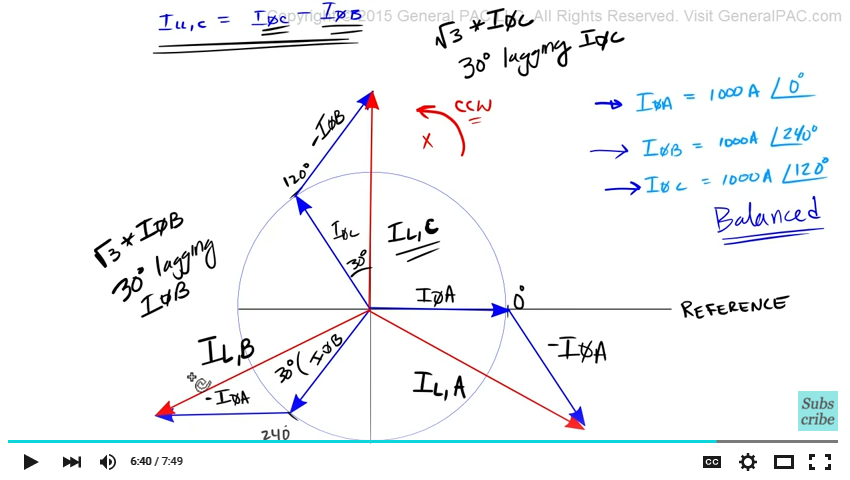We see that the line current have the same magnitudes. The line current also have 120 degree phase difference each of them. So we have 120 degree phase difference here. 120 degrees difference. And 120 degree phase difference here – okay. And all three of them have the same magnitude. Which is the square root of 3 times the phase current.

In this example, we'll see that the line current is a balanced set of 3 phase currents – okay. And we see very very clearly that the line current lag the phase current by 30 degrees. Now to get the update in when part 6 is going to come out, I highly recommend that you subscribe to this channel, and you can subscribe by clicking on the button on the bottom right corner of this screen. Also visit generalpac.com for additional power system videos. Our goal is to make power system protection, automation, and controls intuitive.

Greetings from the GeneralPAC Team!

We make high-quality Power Systems Video Tutorials on complex topics that are free and open to everyone!  Thank you so much for supporting us through Patreon so can continue doing good and valuable work.

What is Patreon and why do we use it?

Patreon is a fantastic portal that allows our fans and community to make monthly contribution (like Netflix subscription) so we can continue creating high-quality power systems video tutorials. In return, you get access to incredible perks like voting on future topics, getting your questions answered, access to VIP Q/A webinars with the creators of GeneralPAC, and much more! We THANK YOU for supporting us

Why do we need your support?

An incredible amount of time and effort is needed to develop high-quality video tutorials. Each video (Part 1 for example) takes approximately 10 hours to complete which includes learning the concept ourselves, brainstorming creative ways to teach and explain the concepts, writing the script, audio recording, video recording, and editing. It's no wonder why Hundreds-of-Thousands of people have watched, liked, subscribed, and left positive comments on Youtube channel. Your support truly makes all the difference.